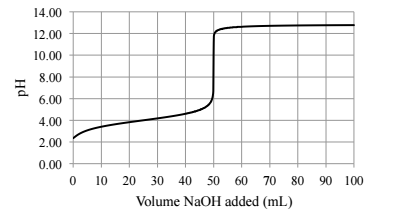# Problem: A titration of 25 mL of a monoprotic acid solution with a 0.1M NaOH solution yielded the following titration curve:a. What was initial molar concentration of the acid? ___________________b. What is the Ka of the acid? ___________________

###### FREE Expert Solution
86% (236 ratings)
###### Problem Details

A titration of 25 mL of a monoprotic acid solution with a 0.1M NaOH solution yielded the following titration curve:

a. What was initial molar concentration of the acid? ___________________

b. What is the Ka of the acid? ___________________What scientific concept do you need to know in order to solve this problem?

Our tutors have indicated that to solve this problem you will need to apply the Acid and Base Titration Curves concept. You can view video lessons to learn Acid and Base Titration Curves. Or if you need more Acid and Base Titration Curves practice, you can also practice Acid and Base Titration Curves practice problems.

What is the difficulty of this problem?

Our tutors rated the difficulty ofA titration of 25 mL of a monoprotic acid solution with a 0....as high difficulty.

How long does this problem take to solve?

Our expert Chemistry tutor, Jules took 3 minutes and 45 seconds to solve this problem. You can follow their steps in the video explanation above.

What professor is this problem relevant for?

Based on our data, we think this problem is relevant for Professor Cotruvo's class at PSU.